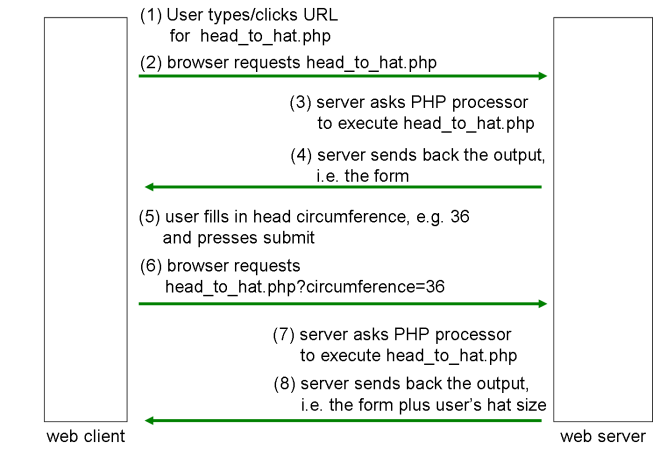# PHP: Self-Processing Pages

Aims:

• to know what a self-processing page is, and how to write one

# Big head

• A person's hat size is obtained by measuring the circumference of the head just above the ears and dividing by π
• Up to now, a program to take in circumferences and output hat sizes would consist of two files, e.g.:
• `head.html`: an HTML web page that contains a form
```<form action="hat.php" method="get">
<input type="text" name="circumference" />
<input type="submit" />
</form>```
• `hat.php`: a PHP script that receives the data, carries out the calculation, and echoes the result into a new web page

# Self-processing page

• We can instead write this using a self-processing page:
• a single PHP script, e.g., `head_to_hat.php`
• when requested without user data, it outputs the form
• when requested with user data, it receives the data, carries out the calculation, and outputs the form again but with the result included

# Self-processing page# Pseudocode

```
if the user is requesting the form for the first time
{
output a web page containing the form;
}
else // i.e. s/he has requested it previously, has filled it in,
// has pressed submit, and is sending us data
{
calculate the person's hat size;
output a web page containing the form and the hat size;
}```

# A useful function

• Class exercise: It is worth defining a function to output the form. Why?
• Note the trick for putting the name of this file as the value for the `action` attribute
```function output_form()
{
echo "<form action=\"{\$_SERVER['PHP_SELF']}\" method=\"get\">";
output_textfield('circumference', 'Circumference: ', 'circumference', 4, 4, '', false);
output_submit_button('Submit');
echo "</form>";
}```

# Another useful function

• We need to be able to distinguish whether the user is requesting the form for the first time (hence, not submitting any data) from the case where s/he has seen the form, filled it in, pressed submit, and sent us data
• Here, as a function, is one of the many ways of checking for this (how it works will be explained in the lecture):
```function is_initial_request()
{
return ! isset(\$_GET['submit']);
}```

# Class exercises

1. Write the rest of the `head_to_hat.php` script
2. If you wanted to validate the user's data, what changes would you make?

# A small modification

• In fact, it might be nice to display the hat size in a text field, instead of as a paragraph under the form
• Class exercise: What changes would you make?